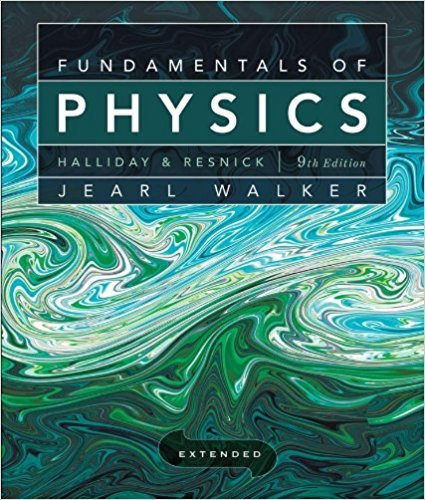×
Log in to StudySoup
Get Full Access to Fundamentals Of Physics Extended - 9 Edition - Chapter 24 - Problem 5
Join StudySoup for FREE
Get Full Access to Fundamentals Of Physics Extended - 9 Edition - Chapter 24 - Problem 5

Already have an account? Login here
×
Reset your password

# Figure 24-25 shows three paths along which we can move theISBN: 9780470469088 189

## Solution for problem 5 Chapter 24

Fundamentals of Physics Extended | 9th Edition

• Textbook Solutions
• 2901 Step-by-step solutions solved by professors and subject experts
• Get 24/7 help from StudySoup virtual teaching assistantsFundamentals of Physics Extended | 9th Edition

4 5 1 411 Reviews
16
1
Problem 5

Figure 24-25 shows three paths along which we can move the positively charged sphere A closer to positively charged sphere B, which is held fixed in place. (a) Would sphere A be moved to a higher or lower electric potential? Is the work Fig.24-25 Question 5. done (b) by our force and (c) by the electric field due to B positive, negative, or zero? (d) Rank the paths according to the work our force does, greatest first.

Step-by-Step Solution:
Step 1 of 3

• Graphs o Velocityvs.TimeGraph § Straighthorizontallinemeans“constantvelocity” v t § Straightslopedlinemeans“constantacceleration”(accelerationisa changeinvelocity) v t o Positionvs.TimeGraph § Straighthorizontallinemeans“atrest” x

Step 2 of 3

Step 3 of 3

##### ISBN: 9780470469088

The answer to “Figure 24-25 shows three paths along which we can move the positively charged sphere A closer to positively charged sphere B, which is held fixed in place. (a) Would sphere A be moved to a higher or lower electric potential? Is the work Fig.24-25 Question 5. done (b) by our force and (c) by the electric field due to B positive, negative, or zero? (d) Rank the paths according to the work our force does, greatest first.” is broken down into a number of easy to follow steps, and 77 words. This full solution covers the following key subjects: . This expansive textbook survival guide covers 37 chapters, and 3413 solutions. Fundamentals of Physics Extended was written by and is associated to the ISBN: 9780470469088. This textbook survival guide was created for the textbook: Fundamentals of Physics Extended, edition: 9. The full step-by-step solution to problem: 5 from chapter: 24 was answered by , our top Physics solution expert on 12/27/17, 08:15PM. Since the solution to 5 from 24 chapter was answered, more than 251 students have viewed the full step-by-step answer.

Unlock Textbook Solution

Enter your email below to unlock your verified solution to:

Figure 24-25 shows three paths along which we can move the